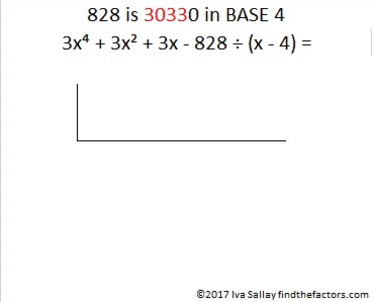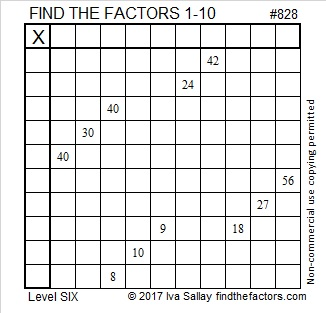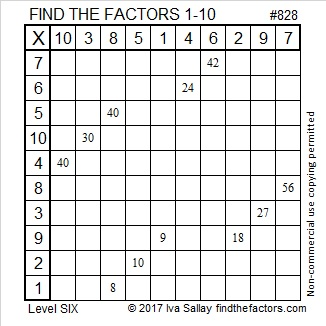# 828 Try Synthetic Division on These Special Polynomials

828 is the sum of consecutive prime numbers 409 and 419.

828 has a lot of factors so I decided to use it in my examples of synthetic division. What are the factors of 828?

• 828 is a composite number.
• Prime factorization: 828 = 2 × 2 × 3 × 3 × 23, which can be written 828 = 2² × 3² × 23
• The exponents in the prime factorization are 2, 2 and 1. Adding one to each and multiplying we get (2 + 1)(2 + 1)(1 + 1) = 3 × 3 × 2 = 18. Therefore 828 has exactly 18 factors.
• Factors of 828: 1, 2, 3, 4, 6, 9, 12, 18, 23, 36, 46, 69, 92, 138, 207, 276, 414, 828
• Factor pairs: 828 = 1 × 828, 2 × 414, 3 × 276, 4 × 207, 6 × 138, 9 × 92, 12 × 69, 18 × 46 or 23 × 36
• Taking the factor pair with the largest square number factor, we get √828 = (√36)(√23) = 6√23 ≈ 28.774989.Synthetic division is taught in many schools in the United States, but in other places in the world it typically is not taught at all. Although I prefer the area model for dividing polynomials, I still like synthetic division. I disagree with those few people who describe it as a mostly useless trick that isn’t worth learning. Yes, its usefulness is limited, but when it can be used, it can be absolutely wonderful. Personally, for many years I always use synthetic division when dividing polynomials by (x-a) or (x+a) where a is any whole number. (If a is a fraction, synthetic division can still be done, but it might not be much fun.)

What are some of the advantages of using synthetic division?

• If you had a polynomial where x is raised to several different powers, such as x⁹ + x⁸ + x⁷ + x⁶ + x⁵ + x⁴ + x³ + x² + x – 8, you would only have to write 1 1 1 1 1 1 1 1 1 -8 to perform the algorithm. That could prevent writer’s cramp if the polynomial is quite long. Ha ha. Seriously, less writing often means fewer chances for mistakes.
• Instead of needing 9×2 lines to do long division for the problem, only three total lines are needed. That saves paper.
• Using a instead of (x-a) or -a instead of (x+a) in the algorithm means we use addition instead of subtraction to find the quotient. Most people make fewer mistakes adding numbers than they do subtracting. Fewer mistakes means less frustration and less erasing.

Before we can do synthetic division we need to write some polynomials. Since this is my 828th post, I will write some polynomials based on the following chart, and they will be very special polynomials!The numbers in bold print end in a zero because the corresponding base number is a factor of 828. For base 11 or greater, sometimes a digit is represented by a letter of the alphabet. The key to translating those letters to the corresponding number in base 10 is A = 10, B = 11, C = 12, D = 13, E = 14, F = 15, G = 16, H = 17, I = 18, J = 19, K = 20, L = 21, M = 22. This chart goes to BASE 28 because √828 ≈ 28.77.

We can write a special polynomial using the digits given from a desired base. The last digit for these special polynomials will be replaced with -828, but as you will see, that original last digit will not be forgotten.

Because 828 is 30330 in BASE 4, let’s use that information as our first example:

• The digits 30330 make the polynomial 3x⁴ + 0x³ + 3x² + 3x -828.
• The digits 3 0 3 3 -828 will be used as the coefficients in our synthetic division algorithm.
• BASE 4 will be seen in the divisor (x – 4) and as “4” in the algorithm.

Now watch as this gif uses synthetic division to find the quotient.make science GIFs like this at MakeaGif

The remainder is zero, and the last digit of 30330 is zero. From the remainder theorem we also know that 3(4⁴) + 3(4²) + 3(4) -828 = 0.

It turns out we can know what the remainder is for each of these special polynomials BEFORE we do any dividing! The remainder will be the last digit times negative one. That does not usually happen when we use synthetic division on a polynomial, but it will always happen on these special polynomials!

Here are a four more examples of writing one of these special polynomials and dividing it using synthetic division. Try writing the rest of the problems using some of the other bases and doing the division yourself, too.Now for today’s Find the Factors puzzle:Print the puzzles or type the solution on this excel file: 10-factors-822-828

It’s not an easy puzzle! If you get stumped, here is the logic I used to solve it:

1. Clue 27 will use a 3, so clue 9 cannot be 3×3. Thus, clues  9 and 18 will put 9 in the first column and 1 and 2 in the top row.
2. Can both 40’s be 4×10? No, because that would use both 10’s, and make the 8 and the 18 use both 2’s. That would mean that clue 10 could not be 10×1 or 2×5.
3. So 56 and one of the 40’s will use both 8’s. That means 24 has to use 4 and 6. Thus 24 and 42 will use both 6’s, so 30 will be 10×3.
4. We know one of the 40’s is 4×10, but we don’t know which one. Nevertheless, we know that its 4 will be in the first column because its 10 cannot be. Since 24 must use 4 and 6, its 4 must be in the top row above the 24.

This table shows the rest of the logic I used:That was pretty complicated, so here’s where all the factors go, too. 🙂